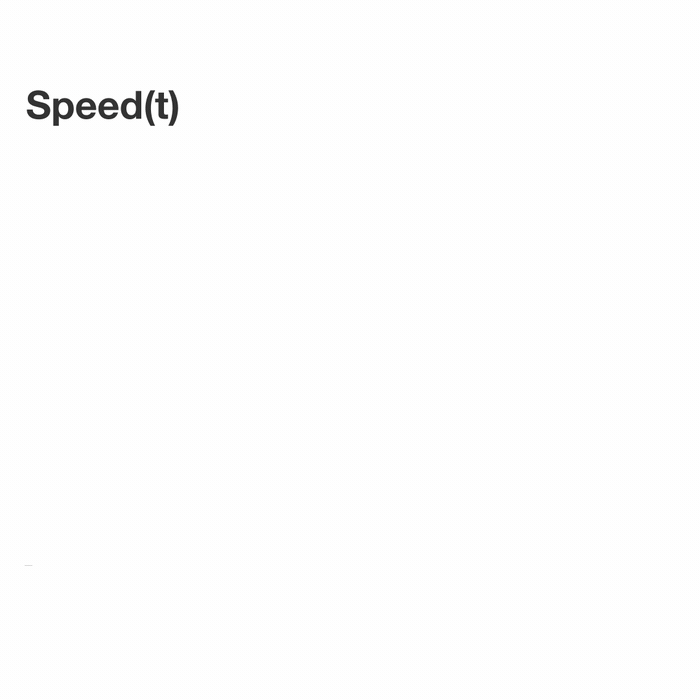### Integral Calculus

When speed changes over time, it is difficult to determine the distance traveled. But we can get a good approximation by dividing the time interval into a large number of pieces and assuming the speed remains constant over each individual period. Then, in each piece, we multiply the change in time by the speed at the start of that interval and sum the products.We’ll call dividing into $n$ intervals an $n$-approximation. So the above shows a 5-approximation of the distance traveled.

# Calculating Distance

A car accelerates with constant acceleration from 0 mph to 60 mph over a 3-hour period.

If we divide this time interval into three intervals (i.e. using a 3-approximation), what are the speeds at the start of the intervals?

# Calculating Distance

The same car still accelerates at a constant rate from 0 mph to 60 mph over a 3-hour period.

Let $d_1$ be a 3-approximation for the distance traveled (i.e. divide the 3-hour period into three smaller time intervals and assume constant speed on them); similarly, let $d_2$ be a 60-approximation (i.e. divide into 60 smaller time intervals) for the distance traveled over the whole 3-hour period.

What are $d_1$ and $d_2?$

(Note we are still taking the speed at the start of each interval.)

# Calculating Distance

Now suppose there is a function that tells us the speed of the car at any given time: in other words, $f(t)$ represents the speed of the car at time $t$. The time interval $[a,b]$ is split into 4 smaller intervals:In terms of $f$, what are the speeds at the beginning of these smaller intervals?

$1. \, \, \, \begin{cases}\ &f(a),\ f\left(a + \frac{b-a}{4}\right),\\ & f\left(a + 2 \cdot \frac{b-a}{4}\right),\ f\left(a + 3 \cdot \frac{b-a}{4}\right)\end{cases}$

$2.\, \, \, \begin{cases} & f(a),\ f\left(a + \frac{b-a}{3}\right),\\ & f\left(a + 2 \cdot \frac{b-a}{3}\right),\ f(b) \end{cases}$

$3.\, \, \, \begin{cases} & f\left(a + \frac{b-a}{4}\right),\ f\left(a + 2 \cdot \frac{b-a}{4}\right),\\ & f\left(a + 3 \cdot \frac{b-a}{4}\right),\ f(b) \end{cases}$

$4.\, \, \, \begin{cases} & f\left(a + \frac{b-a}{5}\right),\ f\left(a + 2 \cdot \frac{b-a}{5}\right),\\ & f\left(a + 3 \cdot \frac{b-a}{5}\right),\ f\left(a + 4 \cdot \frac{b-a}{5}\right) \end{cases}$

# Calculating Distance

Again the car’s speed at time $t$ is $f(t)$. Over the time interval $[a,b]$, what is the 100-approximation (i.e. splitting the time into 100 intervals) to the distance traveled?

# Calculating Distance

In the last problem, we saw that the 100-approximation for distance travelled over the time period $t \in [a,b]$ is

$\frac{b-a}{100}\sum_{i=0}^{99}f\left(a + i \cdot \frac{b-a}{100}\right).$

Generalizing, the $n$-approximation is similarly

$\frac{b-a}{n}\sum_{i=0}^{n-1}f\left(a + i \cdot \frac{b-a}{n}\right).$

This is called a Riemann sum, which for now we’ll write as $\text{RS}(f, a, b, n)$ $($meaning the $n$-approximation of the distance travelled over the time interval $[a,b]$ with speed function $f).$ The idea is that the "true" distance should be a limit of these approximations as $n$ goes to infinity.

In the coming quizzes, we’ll see how the Riemann sum appears not just with distance but in more and more seemingly unrelated places. Then we’ll build up tools to easily evaluate all of these sums!

×